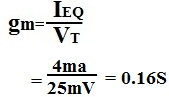﻿ How to Calculate Gm of a Transistor

# How to Calculate gm of a Transistor

Calculating gm is very important when doing AC analysis of a transistor circuit. GmVπ represents the gain of the AC current signal of the transistor after it undergoes amplification.

Gm is a measure of the conductance of a component. It is measured in a unit called siemens(S).

The formula for calculating gm is:VT is the thermal voltage of a transistor; at room temperature, the value is approximately 25mV. The current, IEQ, is obtained by doing DC analysis of the transistor.

Example
Let's suppose that a transistor circuit has a current IEQ of 4ma. Let's now calculate gm.